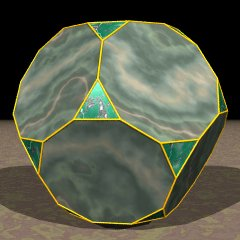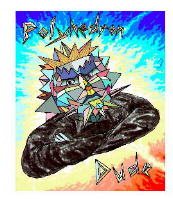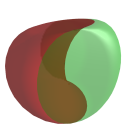Acronym tid
TOCID symbol tD
Name truncated dodecahedron

`© ©`
Vertex figure [3,102]
Vertex layers
 Layer Symmetry Subsymmetries o3o5o o3o . o . o . o5o 1 o3x5x o3x .{3} first o . xedge first . x5x{10} first 2 o3F . x . F . o5F 3 x3V . F . V . o5V 4 F3F . B . F . x5F 5a B3x . V . B . F5x 5b C . x 6a V3F . F . C . V5o 6b D . o 7 F3V . x . D . F5o 8a x3B . F . C . x5xopposite {10} 8b D . o 9a F3F . V . B 9b C . x 10 V3x . B . F 11 F3o . F . V 12 x3o .opposite {3} x . F 13 o . xopposite edge
(F = ff = f+x, V = uf = 2f, B = fff = 2f+x, C = uff = 2f+2x, D = ff.sqrt(5) = 3f+x)
General of army (is itself convex)
Colonel of regiment (is itself locally convex – no other uniform polyhedral members)
Dihedral angles
• between {3} and {10}:   arccos(-sqrt[(5+2 sqrt(5))/15]) = 142.622632°
• between {10} and {10}:   arccos(-1/sqrt(5)) = 116.565051°
Confer
Grünbaumian relatives:
2tid
variations:
a3b5c   o3(-x)5f
related Johnson solids:
autid   pabautid   mabautid   tautid
ExternalAs abstract polytope tid is isomorphic to quit gissid, thereby replacing decagons by decagrams.

Incidence matrix according to Dynkin symbol

```o3x5x

. . . | 60 |  2  1 |  1  2
------+----+-------+------
. x . |  2 | 60  * |  1  1
. . x |  2 |  * 30 |  0  2
------+----+-------+------
o3x . |  3 |  3  0 | 20  *
. x5x | 10 |  5  5 |  * 12

snubbed forms: o3β5x, o3x5β, o3β5β
```

```o3/2x5x

.   . . | 60 |  2  1 |  1  2
--------+----+-------+------
.   x . |  2 | 60  * |  1  1
.   . x |  2 |  * 30 |  0  2
--------+----+-------+------
o3/2x . |  3 |  3  0 | 20  *
.   x5x | 10 |  5  5 |  * 12
```